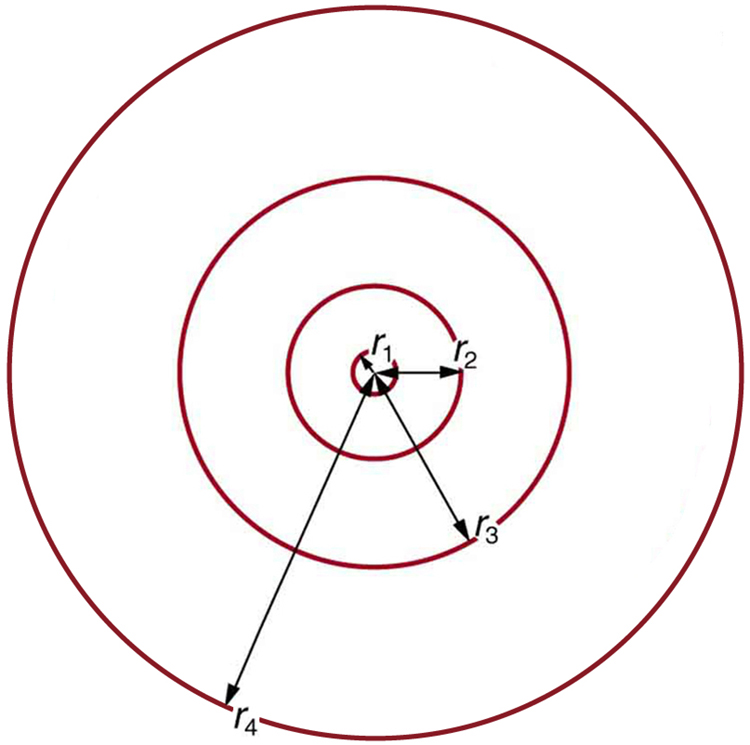# 30.3 Bohr’s theory of the hydrogen atom  (Page 4/14)

 Page 4 / 14
$L={m}_{e}{\text{vr}}_{n}=n\frac{h}{2\pi }\left(n=1, 2, 3,\dots \right)\text{,}$

where $L$ is the angular momentum, ${m}_{e}$ is the electron’s mass, ${r}_{n}$ is the radius of the $n$ th orbit, and $h$ is Planck’s constant. Note that angular momentum is $L=\mathrm{I\omega }$ . For a small object at a radius $r,\phantom{\rule{0.25em}{0ex}}I={\text{mr}}^{2}$ and $\omega =v/r$ , so that $L=\left({\text{mr}}^{2}\right)\left(v/r\right)=\text{mvr}$ . Quantization says that this value of $\text{mvr}$ can only be equal to $h/2,\phantom{\rule{0.25em}{0ex}}2h/2,\phantom{\rule{0.25em}{0ex}}3h/2$ , etc. At the time, Bohr himself did not know why angular momentum should be quantized, but using this assumption he was able to calculate the energies in the hydrogen spectrum, something no one else had done at the time.

From Bohr’s assumptions, we will now derive a number of important properties of the hydrogen atom from the classical physics we have covered in the text. We start by noting the centripetal force causing the electron to follow a circular path is supplied by the Coulomb force. To be more general, we note that this analysis is valid for any single-electron atom. So, if a nucleus has $Z$ protons ( $Z=1$ for hydrogen, 2 for helium, etc.) and only one electron, that atom is called a hydrogen-like atom    . The spectra of hydrogen-like ions are similar to hydrogen, but shifted to higher energy by the greater attractive force between the electron and nucleus. The magnitude of the centripetal force is ${m}_{e}{v}^{2}/{r}_{n}$ , while the Coulomb force is $k\left({\text{Zq}}_{e}\right)\left({q}_{e}\right)/{r}_{n}^{2}$ . The tacit assumption here is that the nucleus is more massive than the stationary electron, and the electron orbits about it. This is consistent with the planetary model of the atom. Equating these,

$k\frac{{\text{Zq}}_{e}^{2}}{{r}_{n}^{2}}=\frac{{m}_{e}{v}^{2}}{{r}_{n}}\left(Coulomb = centripetal\right).$

Angular momentum quantization is stated in an earlier equation. We solve that equation for $v$ , substitute it into the above, and rearrange the expression to obtain the radius of the orbit. This yields:

${r}_{n}=\frac{{n}^{2}}{Z}{a}_{\text{B}}\text{, for allowed orbits}\left(n=1,2,3,\dots \right)\text{,}$

where ${a}_{\text{B}}$ is defined to be the Bohr radius    , since for the lowest orbit $\left(n=1\right)$ and for hydrogen $\left(Z=1\right)$ , ${r}_{1}={a}_{\text{B}}$ . It is left for this chapter’s Problems and Exercises to show that the Bohr radius is

${a}_{\text{B}}=\frac{{h}^{2}}{{4\pi }^{2}{m}_{e}{\text{kq}}_{e}^{2}}=\text{0.529}×{\text{10}}^{-\text{10}}\phantom{\rule{0.25em}{0ex}}\text{m}.$

These last two equations can be used to calculate the radii of the allowed (quantized) electron orbits in any hydrogen-like atom . It is impressive that the formula gives the correct size of hydrogen, which is measured experimentally to be very close to the Bohr radius. The earlier equation also tells us that the orbital radius is proportional to ${n}^{2}$ , as illustrated in [link] .The allowed electron orbits in hydrogen have the radii shown. These radii were first calculated by Bohr and are given by the equation r n = n 2 Z a B size 12{r rSub { size 8{n} } = { {n rSup { size 8{2} } } over {Z} } a rSub { size 8{B} } } {} . The lowest orbit has the experimentally verified diameter of a hydrogen atom.

To get the electron orbital energies, we start by noting that the electron energy is the sum of its kinetic and potential energy:

${E}_{n}=\text{KE}+\text{PE}.$

Kinetic energy is the familiar $\text{KE}=\left(1/2\right){m}_{e}{v}^{2}$ , assuming the electron is not moving at relativistic speeds. Potential energy for the electron is electrical, or $\text{PE}={q}_{e}V$ , where $V$ is the potential due to the nucleus, which looks like a point charge. The nucleus has a positive charge ${\text{Zq}}_{e}$ ; thus, $V={\text{kZq}}_{e}/{r}_{n}$ , recalling an earlier equation for the potential due to a point charge. Since the electron’s charge is negative, we see that $\text{PE}=-{\text{kZq}}_{e}/{r}_{n}$ . Entering the expressions for $\text{KE}$ and $\text{PE}$ , we find

full meaning of GPS system
how to prove that Newton's law of universal gravitation F = GmM ______ R²
sir dose it apply to the human system
prove that the centrimental force Fc= M1V² _________ r
prove that centripetal force Fc = MV² ______ r
Kaka
how lesers can transmit information
griffts bridge derivative
below me
please explain; when a glass rod is rubbed with silk, it becomes positive and the silk becomes negative- yet both attracts dust. does dust have third types of charge that is attracted to both positive and negative
what is a conductor
Timothy
hello
Timothy
below me
why below you
Timothy
no....I said below me ...... nothing below .....ok?
dust particles contains both positive and negative charge particles
Mbutene
corona charge can verify
Stephen
when pressure increases the temperature remain what?
remains the temperature
betuel
what is frequency
define precision briefly
CT scanners do not detect details smaller than about 0.5 mm. Is this limitation due to the wavelength of x rays? Explain.
hope this helps
what's critical angle
The Critical Angle Derivation So the critical angle is defined as the angle of incidence that provides an angle of refraction of 90-degrees. Make particular note that the critical angle is an angle of incidence value. For the water-air boundary, the critical angle is 48.6-degrees.
okay whatever
Chidalu
pls who can give the definition of relative density?
Temiloluwa
the ratio of the density of a substance to the density of a standard, usually water for a liquid or solid, and air for a gas.
Chidalu
What is momentum
mass ×velocity
Chidalu
it is the product of mass ×velocity of an object
Chidalu
how do I highlight a sentence]p? I select the sentence but get options like copy or web search but no highlight. tks. src
then you can edit your work anyway you want
Wat is the relationship between Instataneous velocity
Instantaneous velocity is defined as the rate of change of position for a time interval which is almost equal to zero
AstronomyByByBy OpenStaxBy OpenStaxBy OpenStaxBy Richley CrapoBy Rohini AjayBy Janet ForresterBy OpenStaxBy OpenStaxBy OpenStaxBy